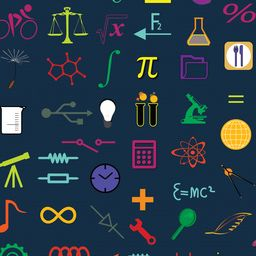Do you want to practice math?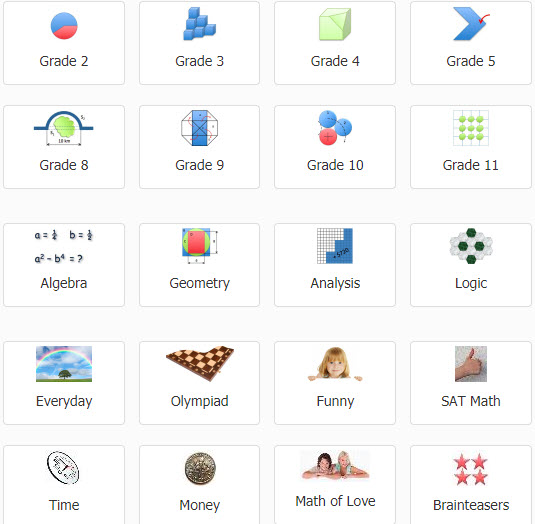### A+ Click Math Problems

Test your math skills with problems that help develop logical reasoning and problem-solving skills.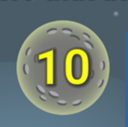### Addition Blast Off

Can you add as fast as a rocket? Test your math skills with Addition Blast Off from NASA.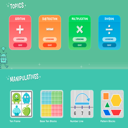### Cool Math for Kids

Play games, practice your math facts, and have fun with numbers. For elementary students.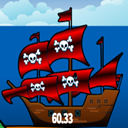### Decimals of the Caribbean

Practice matching numbers with words by blasting treasure ships that sail through the Caribbean with the number of the flag that matches with words on the screen. (If you're at home, you'll need your school's INFOhio username/password. If you don't know it, find it on INFOhio's Get Password page.)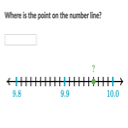### Decimals On the Number Line: Hundredths

Practice identifying decimals to hundredths on a number line using this practice module from Khan Academy.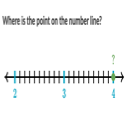### Decimals on the Number Line: Tenths

Practice identifying decimals to tenths on a number line using this practice module from Khan Academy.### Figure This!

Take a real-world math challenge! Mathematical challenges for middle-school students.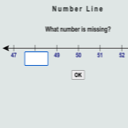### Number Line--What Number is Missing?

Work with number lines to fill in missing numbers.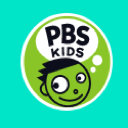### PBS Kids Math Games

Play math games with your favorite characters.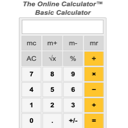### The Online Calculator

Basic online calculator with a 10-digit keypad and four functions to add, subtract, multiply, and divide numbers. Includes basic handheld calculator functions for square, square root, percent, sign change, Pi, and memory.